### Home > CALC > Chapter 9 > Lesson 9.1.3 > Problem9-42

9-42.
1. No Calculator! Evaluate the following limits. Homework Help ✎

1.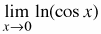2.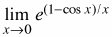3.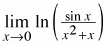4.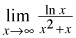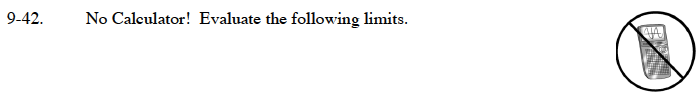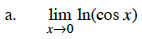What is cos(0)?$\text{What is }\lim_{x\rightarrow0}\frac{1-\cos(x)}{x}\text{?}$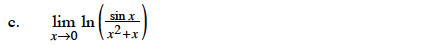$\text{This is }\lim_{x\rightarrow0}\text{ }\ln\Big(\frac{\sin(x)}{x}\cdot\frac{1}{x+1}\Big).$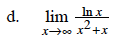Use l'Hôpital's Rule.